# Two-term congruence

(diff) ← Older revision | Latest revision (diff) | Newer revision → (diff)

binomial congruence, power congruence

An algebraic congruence of the form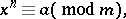(1)

where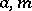are coprime integers andis a natural number. If the congruence (1) is solvable,is called an-th power residue modulo; otherwise,is an-th power non-residue modulo.

The problem of solvability of a two-term congruence to a composite moduluscan be reduced to the study of the corresponding problem to a prime modulus(cf. Congruence (in algebra)). For the prime modulus power congruence problem there is a solvability criterion, due to L. Euler: For the congruence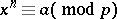to be solvable, it is necessary that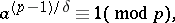whereis the greatest common divisor of the numbersand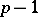; if this condition is met, the congruence in question has exactlysolutions.

It follows immediately from Euler's criterion that the numbershave exactly-th power residues andnon-residues modulo.

The converse problem is much more complicated: To find all modulito which a given numberis a residue (or a non-residue) of power. It was established by Euler that the solvability or non-solvability of the congruence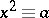(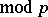) depends on whether or not the prime modulusforms part of certain arithmetical progressions. A rigorous proof of this result was given first by C.F. Gauss in 1801 (see  and Gauss reciprocity law; Quadratic reciprocity law). Gauss further noted that a complete solution of the problem foris only possible if the ring of rational integers is extended somewhat. Thus, in establishing the reciprocity law for biquadratic residues he was forced to extend the ring of rational integers to the ring of complex integers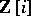. The solvability or non-solvability of the biquadratic congruence() in the ringfor a givenwill depend on the value of the residue of the numberto some constant modulusof the ring.

A new stage in the study of two-term congruences and their applications to other theoretical problems was initiated by I.M. Vinogradov, who showed in 1914 that the numberof quadratic residues to the prime modulusamong the numbers,, is given by the formula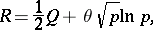where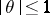. A similar result was subsequently obtained by Vinogradov for a more general problem on the number of solutions of the congruence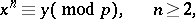whenruns through an incomplete system of residues.

How to Cite This Entry:
Two-term congruence. Encyclopedia of Mathematics. URL: http://encyclopediaofmath.org/index.php?title=Two-term_congruence&oldid=12675
This article was adapted from an original article by S.A. Stepanov (originator), which appeared in Encyclopedia of Mathematics - ISBN 1402006098. See original article